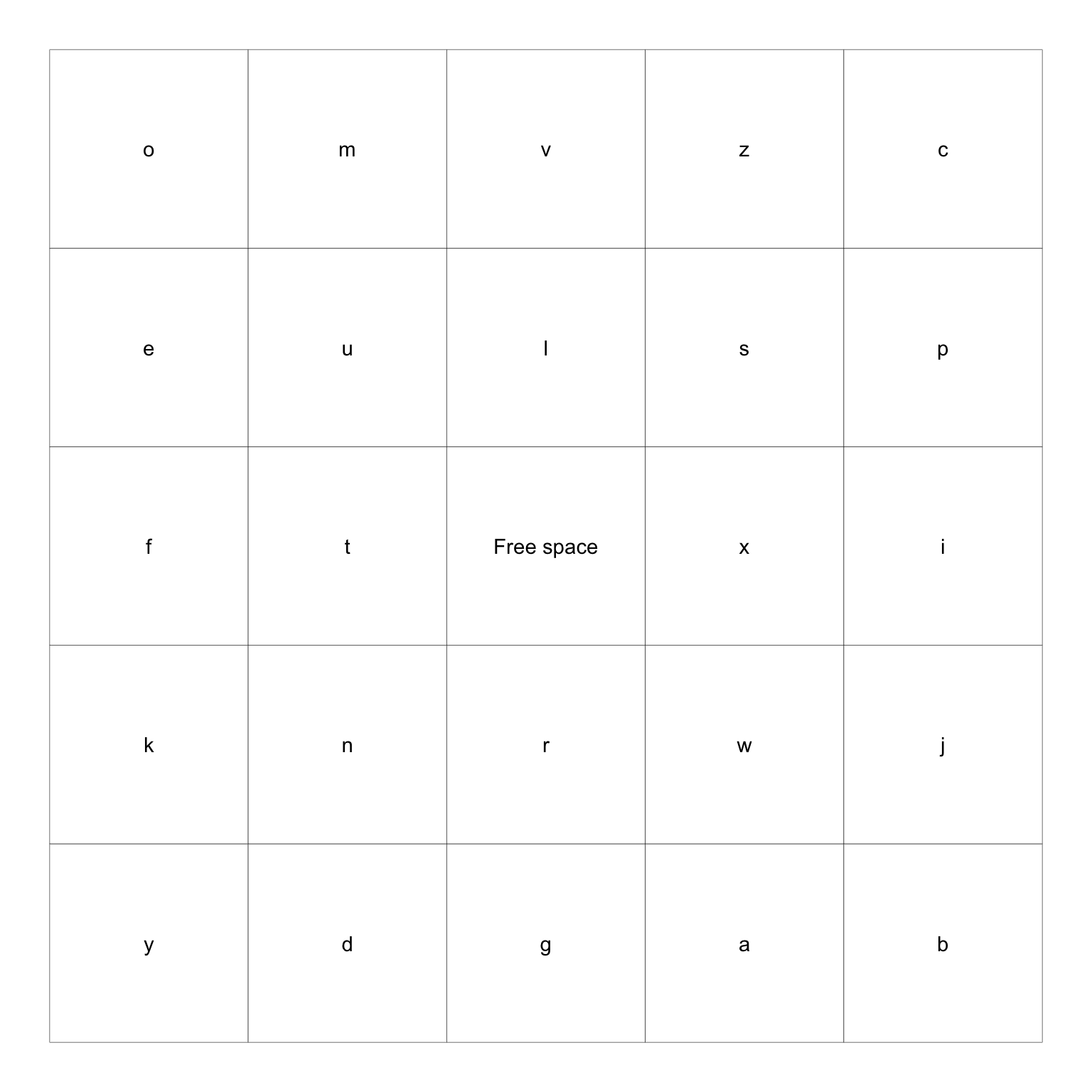library(tidyverse)
# replace this with whatever you're interested in
str <- letters
set.seed(1)
tibble(what = sample(str, 25),
row = rep(1:5, 5),
col = rep(1:5, each = 5)) %>%
mutate(what = ifelse(row == 3 & col == 3,
"Free space", what)) %>%
ggplot(aes(row, col)) +
geom_tile(color = "black", fill = "white") +
geom_text(aes(label = what)) +
coord_fixed() +
theme_void()